出租广告位,需要合作请联系站长

+关注

2021-08(3)

2021-09(11)

# 一、单目测距

## 1.相机标定

https://blog.csdn.net/spw_1201/article/details/78417551

### （1）获取标定板### （2）拍摄图片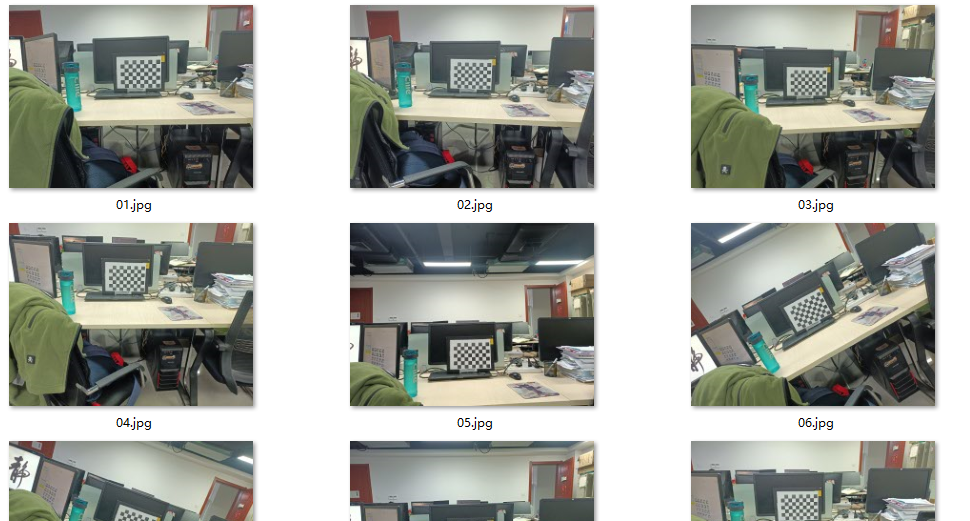### （3）进行标定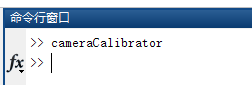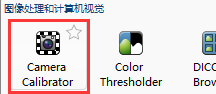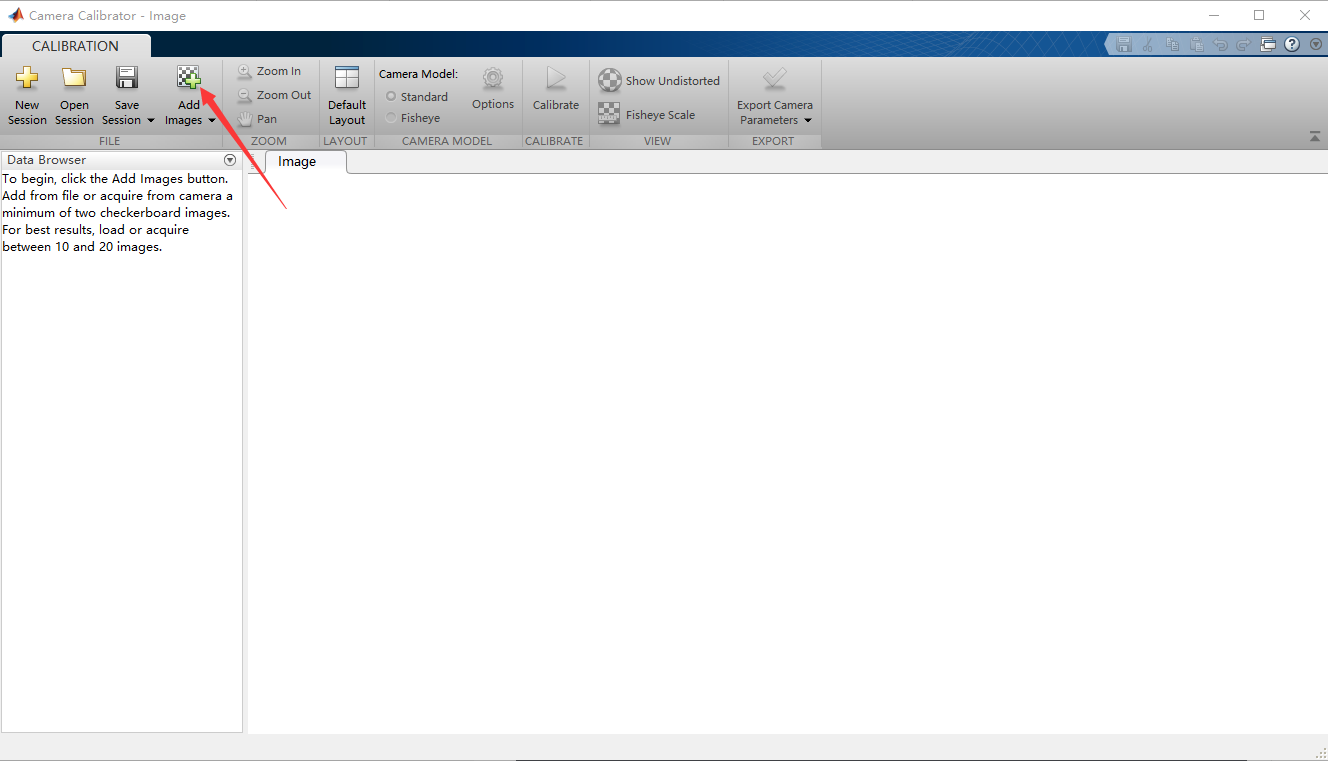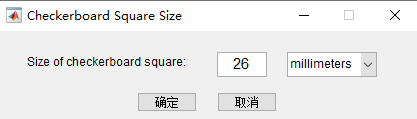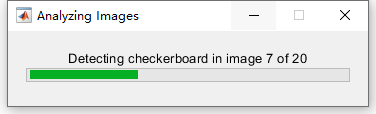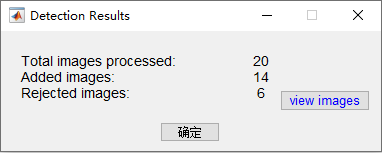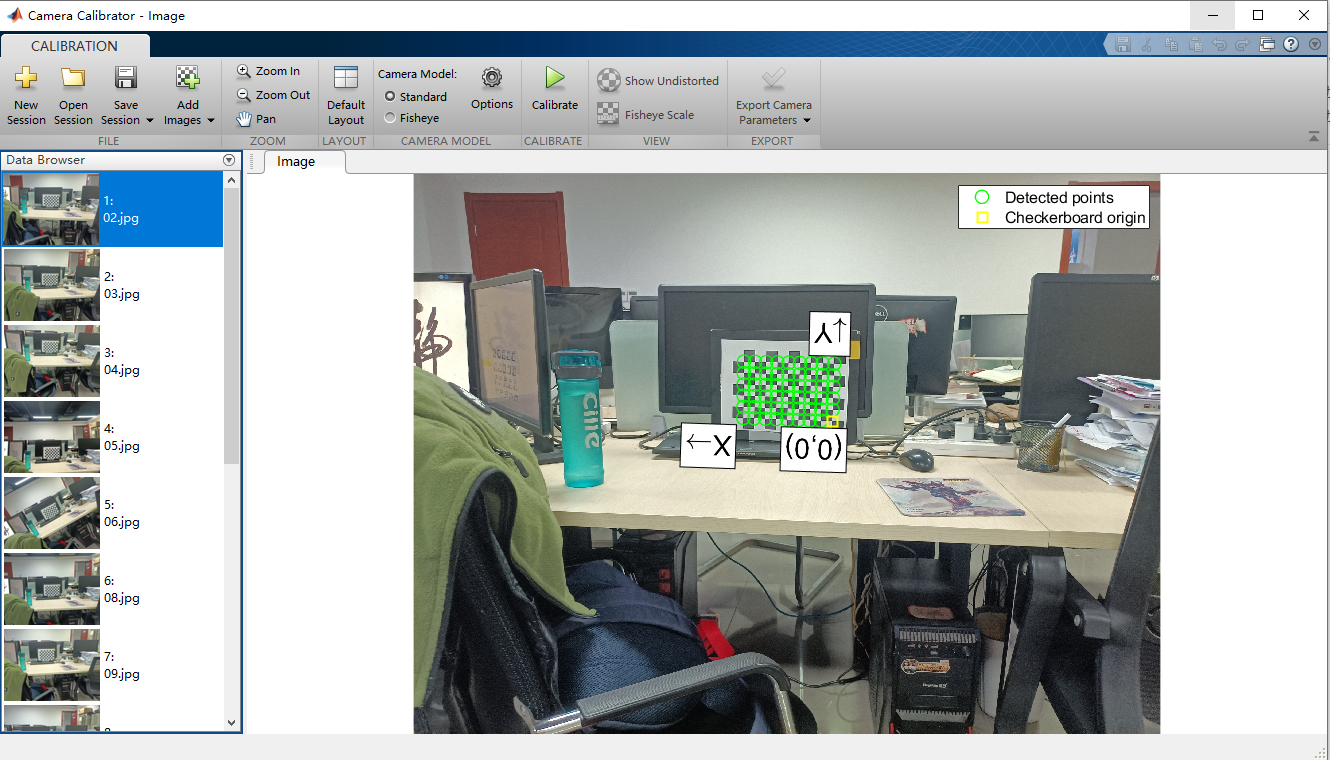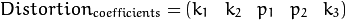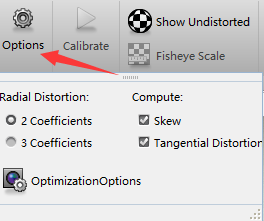1.camera model : standard(标准) fisheye(鱼眼),我的摄像头是标准。

2.options:选中径向畸变:“2 coefficients”并且选择偏差:“Skew“和切向畸变:“Tangential Distortion“

（这些选项根据你的相机进行选择）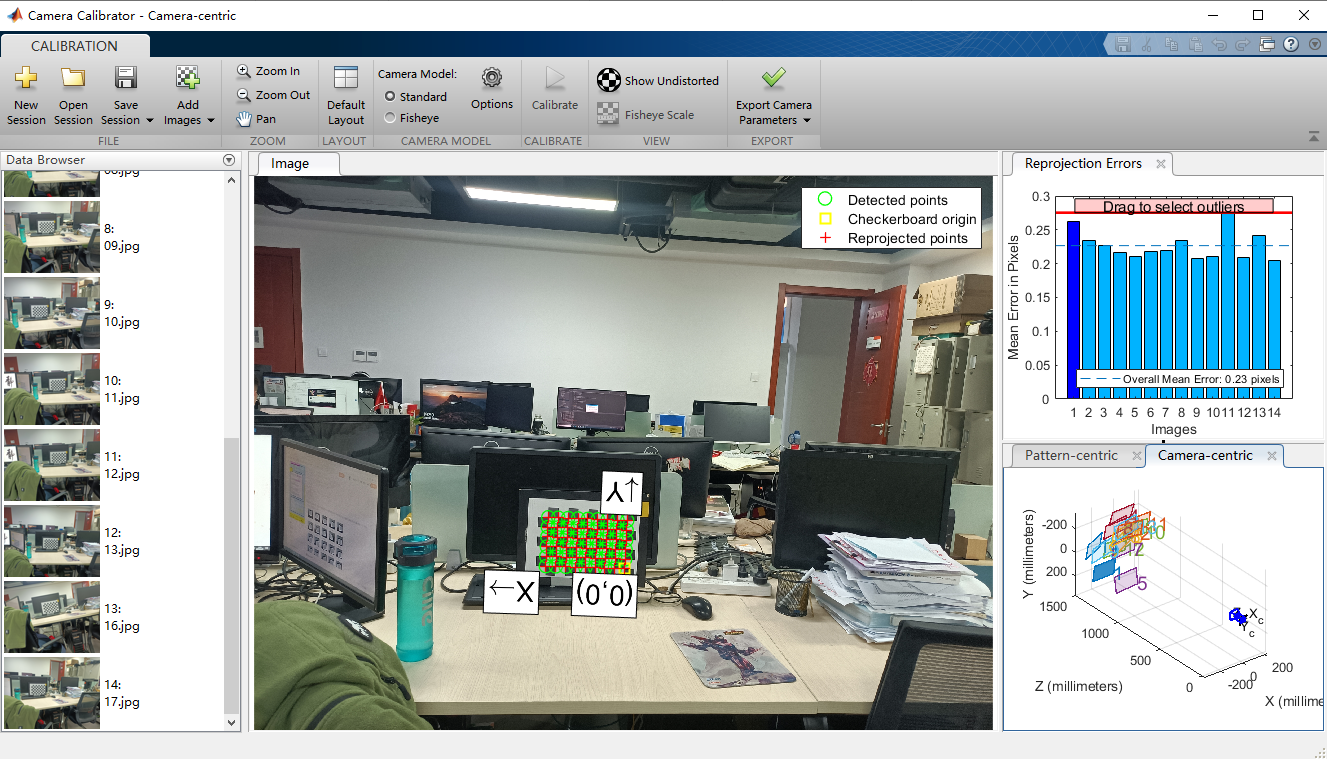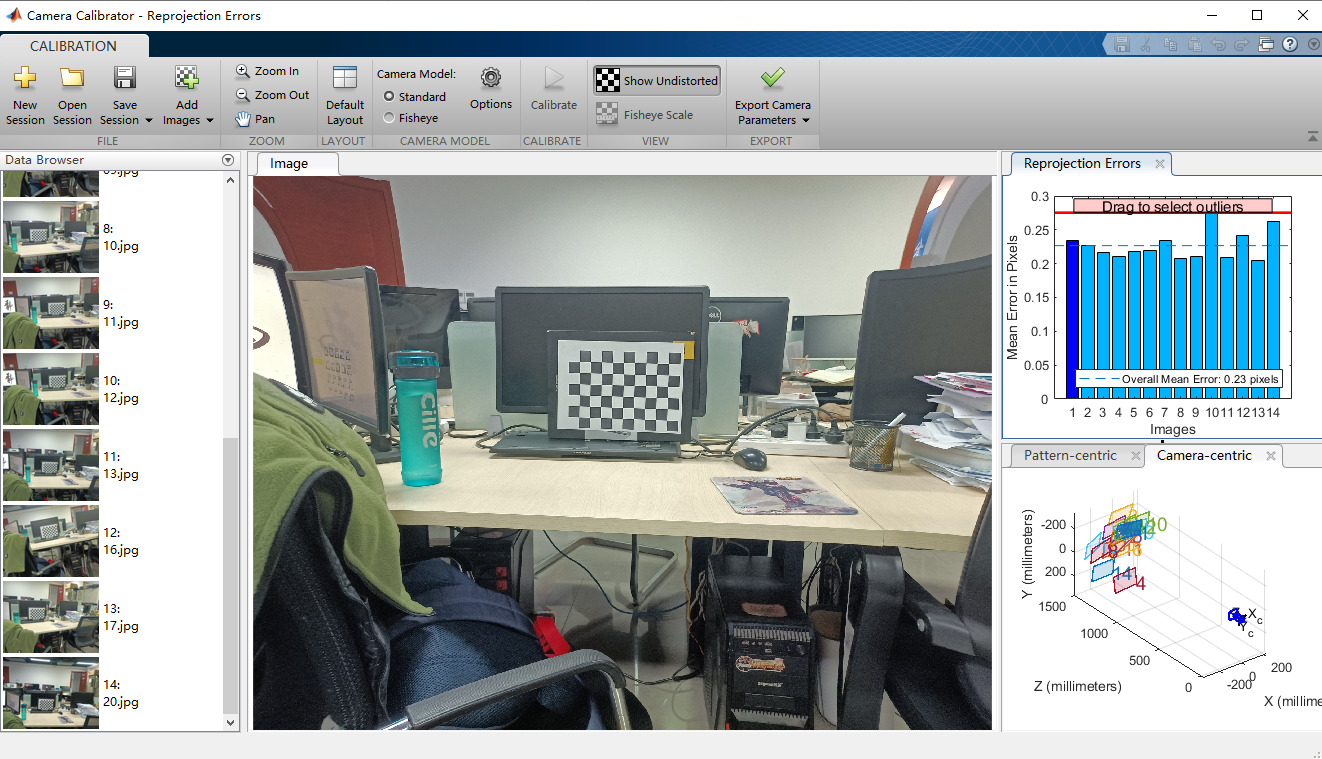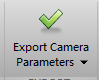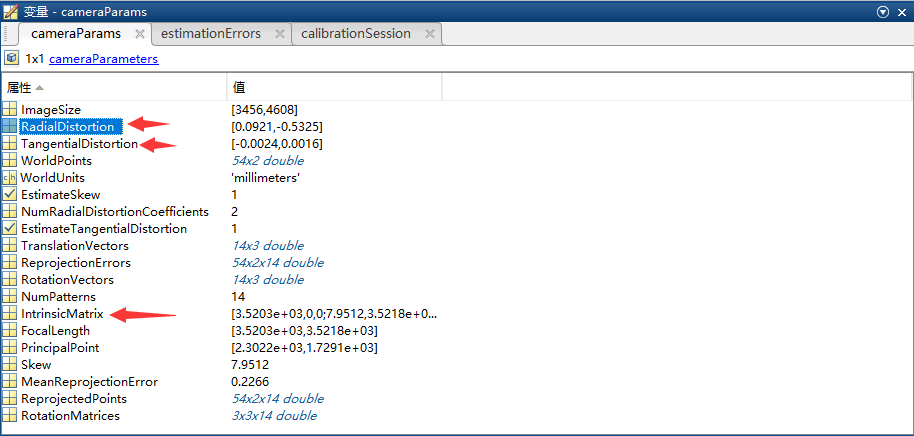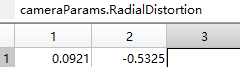TangentialDistortion对应 p1，p2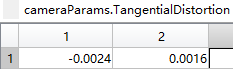IntrinsicMatrix对应 内参数矩阵，注意这个和OpenCV中是转置的关系，注意不要搞错。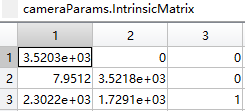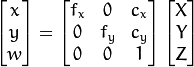FocalLength对应 相机焦距，这个在后面进行测距工作会用到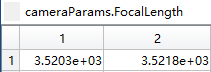### （4）使用opencv查看标定结果

``````import cv2
import numpy as np

#将相机的参数设置好，固定值
#相机内参数矩阵，3*3矩阵
cameraMatrix = np.array([[3.520275278305173e+03, 7.951186274833666, 2.302224660073940e+03],
[0, 3.521771625935550e+03, 1.729056461937039e+03],
[0, 0, 1]])

#相机畸变系数矩阵，5*1矩阵(k1,k2,p1,p2,k3)
distCoeffs = np.array([0.092142020349690, -0.532537876414274, -0.002400984624408, 0.001577353702050, 0])

# 读入原图片
h, w = img.shape[:2]

newCameraMatrix, roi = cv2.getOptimalNewCameraMatrix(cameraMatrix, distCoeffs, (w,h), 1, (w,h), 0)
# 计算无畸变和修正转换关系
mapx, mapy = cv2.initUndistortRectifyMap(cameraMatrix, distCoeffs, None, newCameraMatrix, (w,h), cv2.CV_16SC2)

# 重映射
dst = cv2.remap(img, mapx, mapy, cv2.INTER_LINEAR)

# 调整显示窗口大小
cv2.namedWindow("dst",0);
cv2.resizeWindow("dst", 1268, 952);
cv2.imshow("dst", dst)
#按任意键退出
cv2.waitKey(0)
cv2.destroyAllWindows()
``````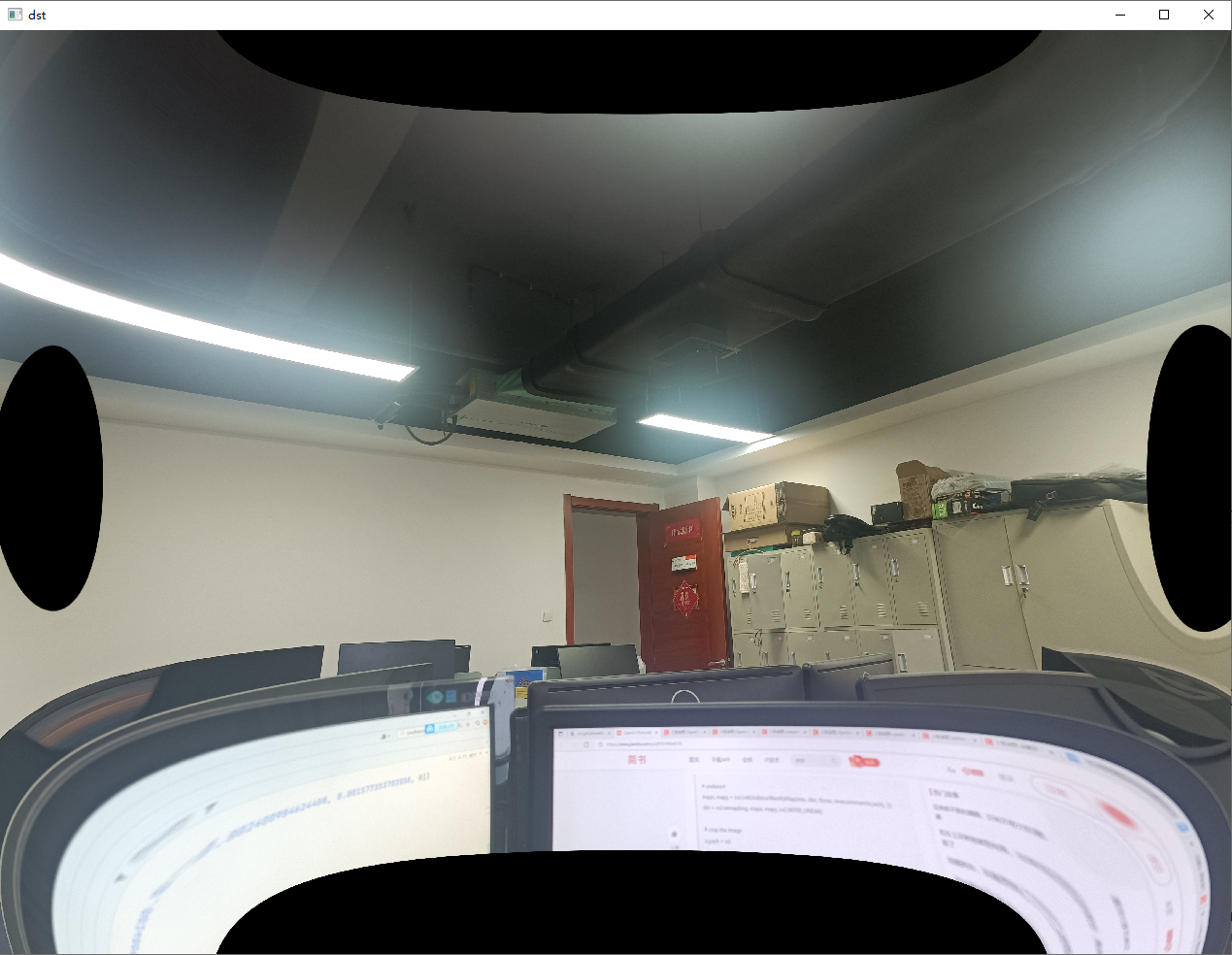## 2.测距

https://blog.csdn.net/m0_37811342/article/details/80394935

F = (P*X) / W

X = (F*W) / P

F我们在上面可以得到：
Fx = 3.520275278305173e+03
Fy = 3.521771625935550e+03

https://www.cnblogs.com/zipeilu/p/6658177.html``````import cv2
import math
import numpy as np

cv2.namedWindow("img",0);
cv2.resizeWindow("img", 1268, 952);
cv2.imshow("img",img) #显示原图像

dst = img.copy()
gray = cv2.cvtColor(img,cv2.COLOR_BGR2GRAY) #转为灰度值图
gaussian = cv2.GaussianBlur(gray, (5, 5), 0, 0) #高斯去噪
cv2.namedWindow("gaussian",0);
cv2.resizeWindow("gaussian", 1268, 952);
cv2.imshow("gaussian", gaussian)

ret, binary = cv2.threshold(gaussian,127,255,cv2.THRESH_BINARY) #转为二值图
cv2.namedWindow("binary",0);
cv2.resizeWindow("binary", 1268, 952);
cv2.imshow("binary", binary)

contours, hierarchy = cv2.findContours(binary,cv2.RETR_LIST,cv2.CHAIN_APPROX_SIMPLE) #寻找轮廓

#n=len(contours)       #轮廓个数
#for i in range(n):
#去除掉根本不可能是要识别的矩形的物体，这个可以在
# if len(contours[i]) >= 4:
#     print(contours[i])
#     length = cv2.arcLength(contours[i], True)  #获取轮廓长度
#     area = cv2.contourArea(contours[i])        #获取轮廓面积
#     print('length['+str(i)+']长度=',length)
#     print("contours["+str(i)+"]面积=",area)
#     cv2.drawContours(dst, contours, i, (0, 0, 255), 3) #绘制轮廓

#通过面积来筛选出我们要识别的矩形
c = max(contours, key=cv2.contourArea)
print(c)
cv2.drawContours(dst, c, -1, (0, 0, 255), 3) #绘制轮廓
#轮廓长度
length = cv2.arcLength(c, True)
#轮廓面积
area = cv2.contourArea(c)
#通过周长和面积计算矩形的高度和宽度
x = length/4 - (math.sqrt(math.pow(length, 2)/16 - area))
y = length/2 - x
print("高度或宽度：", x)
print("高度或宽度：", y)
cv2.namedWindow("dst",0);
cv2.resizeWindow("dst", 1268, 952);
cv2.imshow("dst", dst)
cv2.waitKey()
cv2.destroyAllWindows()
``````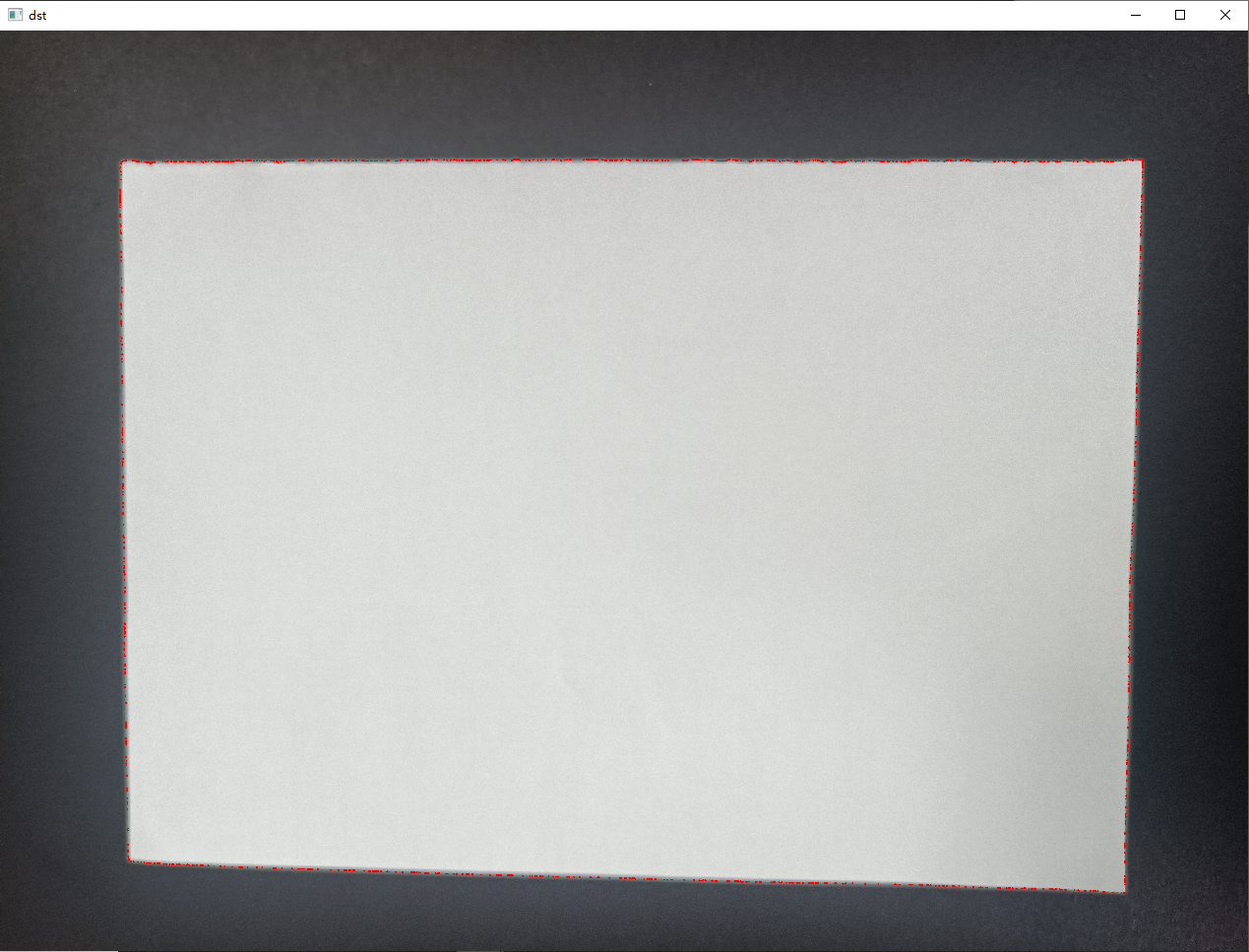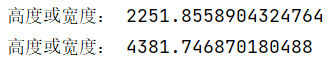（1）通过高度计算：

（2）通过宽度计算：

6 0

pdf(new) 更多>